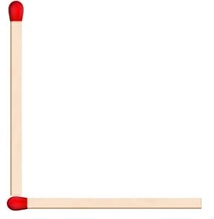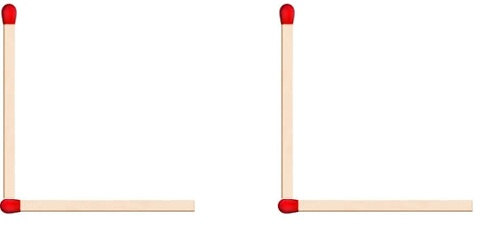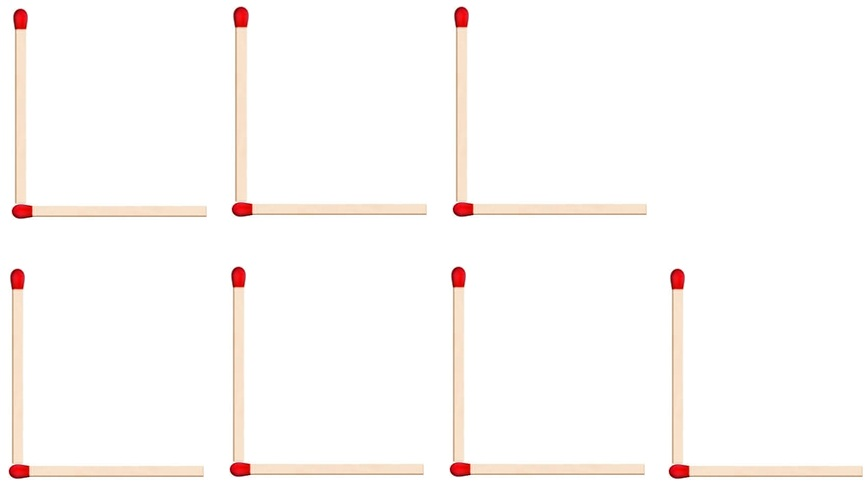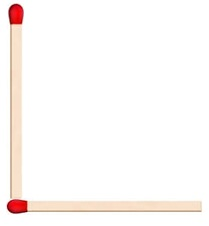Matchstick pattern

Chapter 11 Class 6 Algebra
Concept wise

Suppose we use matchsticks to form letterHere, we used 2 matchsticks

Suppose, we want to make more than one LSo

2 L's require 4 matchsticks

3 L's require 6 matchsticks

4 L's require 8 matchsticks

And so on

So,

Can we say that

Number of matchsticks = 2 × Number of L’s

Writing n = number of L’s

Number of matchsticks = 2nLet’s Check ?

For n = 1,  Number of matchsticks = 2 × 1 = 2

For n = 2,  Number of matchsticks = 2 × 2 = 4

For n = 3,  Number of matchsticks = 2 × 3 = 6

So writing

Number of matchsticks = 2n

Makes our job easier

Suppose we have to make 100 L’s

Number of matchsticks = 2 × 100

= 200

Learn in your speed, with individual attention - Teachoo Maths 1-on-1 Class